Question

# 1) What chemical is the titrant in this experiment? What chemical is the analyte in this experiment? Experi...

1) What chemical is the titrant in this experiment? What chemical is the analyte in this
experiment?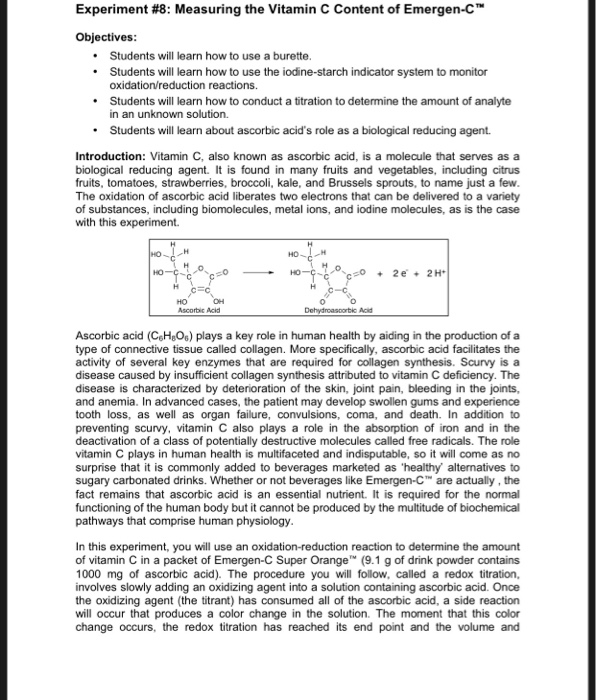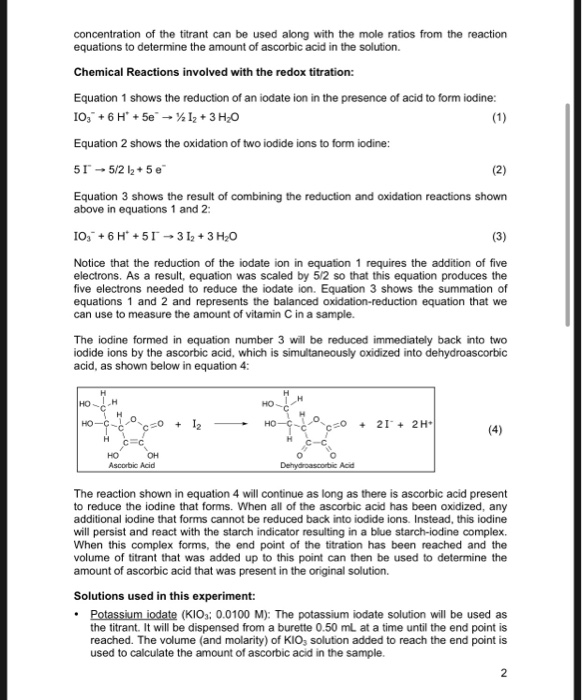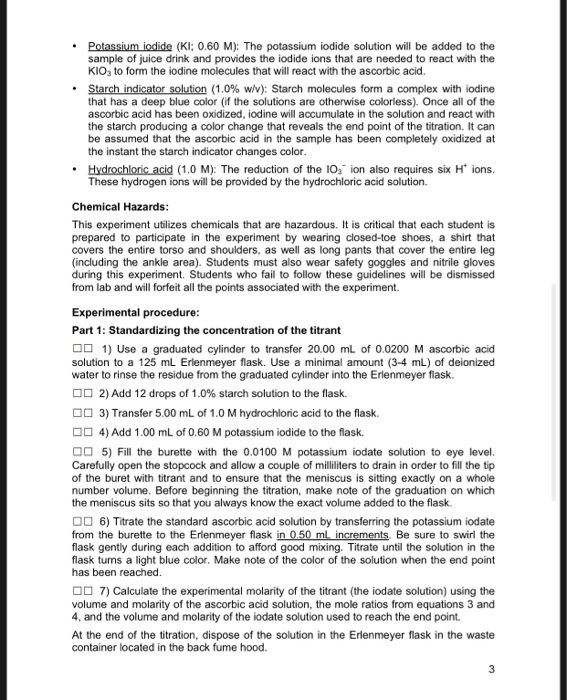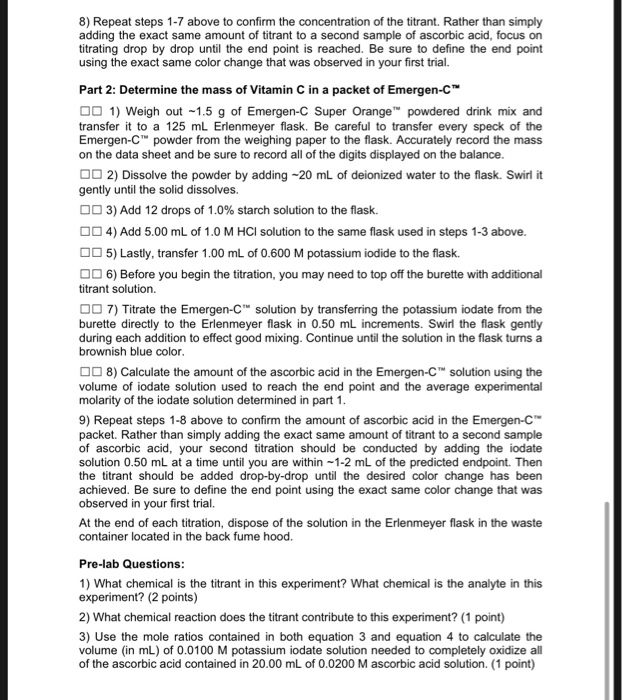Experiment #8: Measuring the Vitamin C Content of Emergen-C™ Objectives: • Students will learn how to use a burette. • Students will learn how to use the iodine starch indicator system to monitor oxidation/reduction reactions. Students will learn how to conduct a titration to determine the amount of analyte in an unknown solution. • Students will learn about ascorbic acid's role as a biological reducing agent. Introduction: Vitamin C, also known as ascorbic acid, is a molecule that serves as a biological reducing agent. It is found in many fruits and vegetables, including citrus fruits, tomatoes, strawberries, broccoli, kale, and Brussels sprouts, to name just a few. The oxidation of ascorbic acid liberates two electrons that can be delivered to a variety of substances, including biomolecules, metal ions, and iodine molecules, as is the case with this experiment. MO- GO HO--- на ЕС HO-200 + 26 2H HC- но он Ascorbic Acid 0 0 Dehydroascorbic Acid Ascorbic acid (C.H.O.) plays a key role in human health by aiding in the production of a type of connective tissue called collagen. More specifically, ascorbic acid facilitates the activity of several key enzymes that are required for collagen synthesis. Scurvy is a disease caused by insufficient collagen synthesis attributed to vitamin C deficiency. The disease is characterized by deterioration of the skin, joint pain, bleeding in the joints, and anemia. In advanced cases, the patient may develop swollen gums and experience tooth loss, as well as organ failure, convulsions, coma, and death. In addition to preventing scurvy, vitamin C also plays a role in the absorption of iron and in the deactivation of a class of potentially destructive molecules called free radicals. The role vitamin C plays in human health is multifaceted and indisputable, so it will come as no surprise that it is commonly added to beverages marketed as 'healthy alternatives to sugary carbonated drinks. Whether or not beverages like Emergen-C™ are actually, the fact remains that ascorbic acid is an essential nutrient. It is required for the normal functioning of the human body but it cannot be produced by the multitude of biochemical pathways that comprise human physiology. In this experiment, you will use an oxidation-reduction reaction to determine the amount of vitamin C in a packet of Emergen-C Super Orange (9.1 g of drink powder contains 1000 mg of ascorbic acid). The procedure you will follow, called a redox titration, involves slowly adding an oxidizing agent into a solution containing ascorbic acid. Once the oxidizing agent (the titrant) has consumed all of the ascorbic acid, a side reaction will occur that produces a color change in the solution. The moment that this color change occurs, the redox titration has reached its end point and the volume and
concentration of the titrant can be used along with the mole ratios from the reaction equations to determine the amount of ascorbic acid in the solution. Chemical Reactions involved with the redox titration: Equation 1 shows the reduction of an iodate ion in the presence of acid to form iodine: 10, +6 +5e 12 + 3 H2O Equation 2 shows the oxidation of two iodide ions to form iodine: 51-5/2 12.5 e Equation 3 shows the result of combining the reduction and oxidation reactions shown above in equations 1 and 2: 10, +6 +51 - 3 12 + 3 H20 Notice that the reduction of the iodate ion in equation 1 requires the addition of five electrons. As a result, equation was scaled by 5/2 so that this equation produces the five electrons needed to reduce the iodate ion. Equation 3 shows the summation of equations 1 and 2 and represents the balanced oxidation-reduction equation that we can use to measure the amount of vitamin C in a sample. The iodine formed in equation number 3 will be reduced immediately back into two lodide ions by the ascorbic acid, which is simultaneously oxidized into dehydroascorbic acid, as shown below in equation 4: HO-ở- t e =0 + 12 - 21° + 2H HO он Ascorbic Acid The reaction shown in equation 4 will continue as long as there is ascorbic acid present to reduce the iodine that forms. When all of the ascorbic acid has been oxidized, any additional lodine that forms cannot be reduced back into iodide ions. Instead, this iodine will persist and react with the starch indicator resulting in a blue starch-iodine complex When this complex forms, the end point of the titration has been reached and the volume of titrant that was added up to this point can then be used to determine the amount of ascorbic acid that was present in the original solution. Solutions used in this experiment: • Potassium iodate (KIO: 0.0100 M): The potassium iodate solution will be used as the titrant. It will be dispensed from a burette 0.50 ml at a time until the end point is reached. The volume (and molarity) of KIO, solution added to reach the end point is used to calculate the amount of ascorbic acid in the sample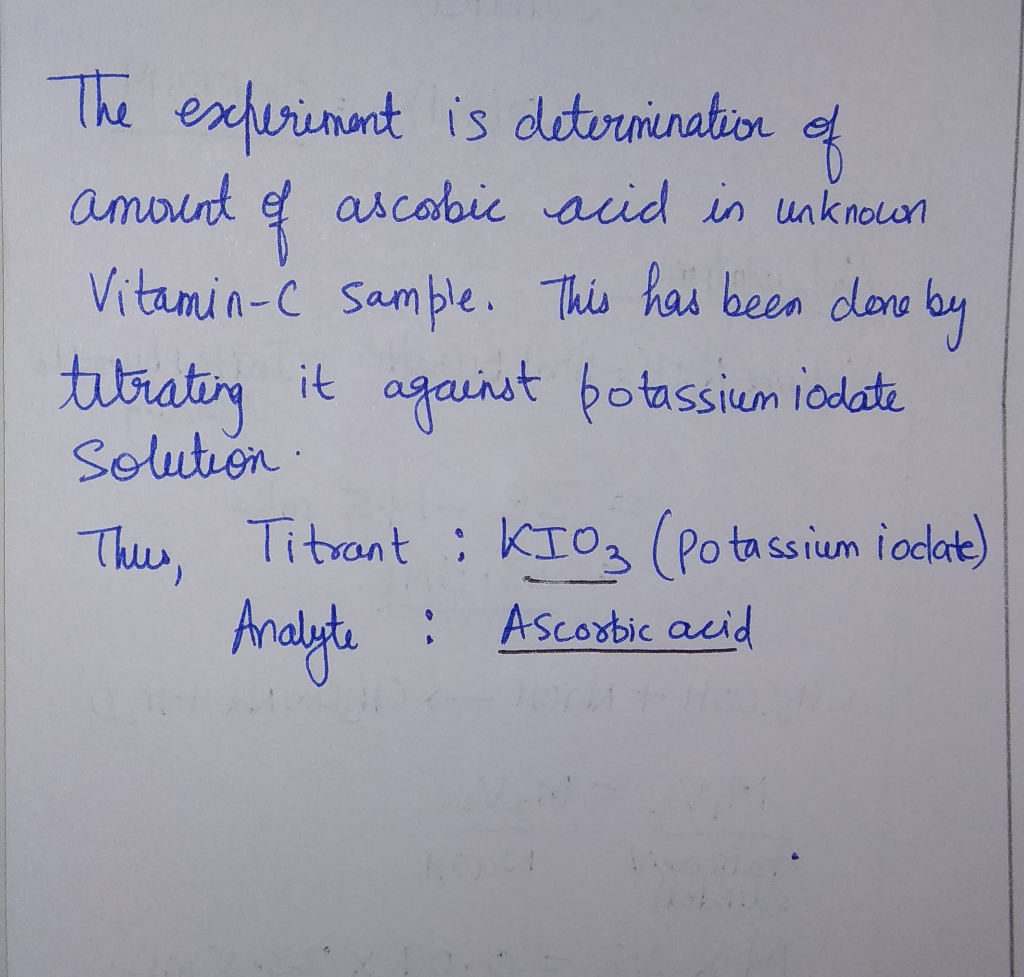#### Earn Coins

Coins can be redeemed for fabulous gifts.

Similar Homework Help Questions
• ### 2) What chemical reaction does the titrant contribute to this experiment? CHEM 1A Experiment #8: Measuring the Vit...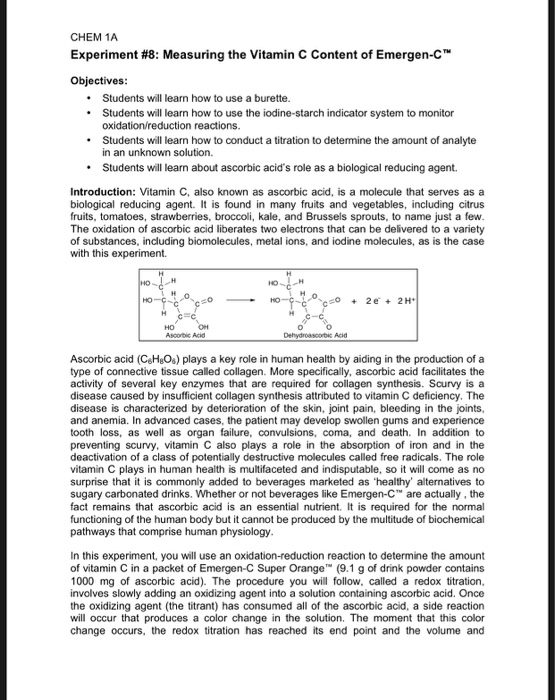2) What chemical reaction does the titrant contribute to this experiment? CHEM 1A Experiment #8: Measuring the Vitamin C Content of Emergen-C™ Objectives: Students will learn how to use a burette. Students will learn how to use the iodine starch indicator system to monitor oxidation/reduction reactions. • Students will learn how to conduct a titration to determine the amount of analyte in an unknown solution. • Students will learn about ascorbic acid's role as a biological reducing agent. Introduction: Vitamin...

• ### 3) Use the mole ratios contained in both equation 3 and equation 4 to calculate the...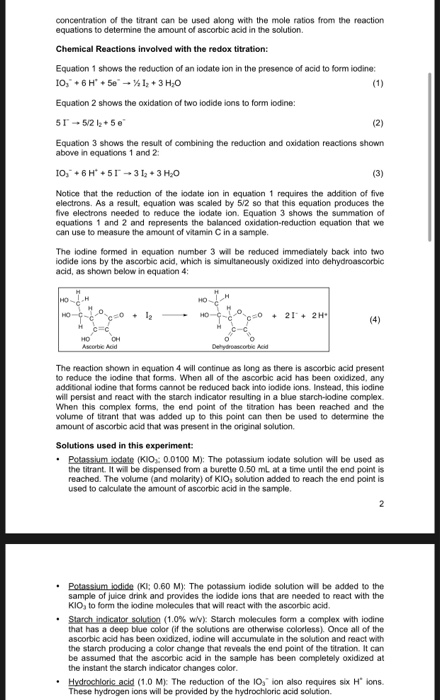3) Use the mole ratios contained in both equation 3 and equation 4 to calculate the volume (in mL) of 0.0100 M potassium iodate solution needed to completely oxidize all of the ascorbic acid contained in 20.00 mL of 0.0200 M ascorbic acid solution. concentration of the titrant can be used along with the mole ratios from the reaction equations to determine the amount of ascorbic acid in the solution Chemical Reactions involved with the redox titration: Equation 1 shows...

• ### Vitamin C in a titration with potassium iodate References Mailings Review View AaBbcode Abccdee AaBbcc No...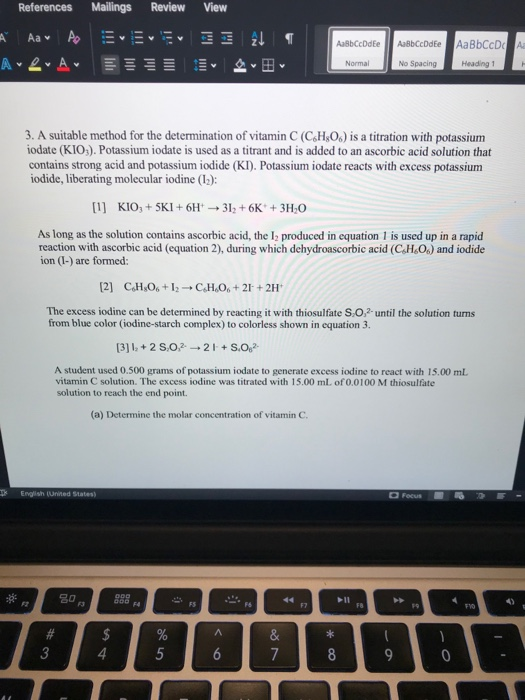Vitamin C in a titration with potassium iodate References Mailings Review View AaBbcode Abccdee AaBbcc No Spacing Heading 1 Normal 3. A suitable method for the determination of vitamin C (C.H.O.) is a titration with potassium iodate (KIO). Potassium iodate is used as a titrant and is added to an ascorbic acid solution that contains strong acid and potassium iodide (KI). Potassium iodate reacts with excess potassium iodide, liberating molecular iodine (12):  KIO, + 5KI + 6H 31, +6K...

• ### Determination of Vitamin C Concentration by Redox Titration An alternative titration method for determining the concentration...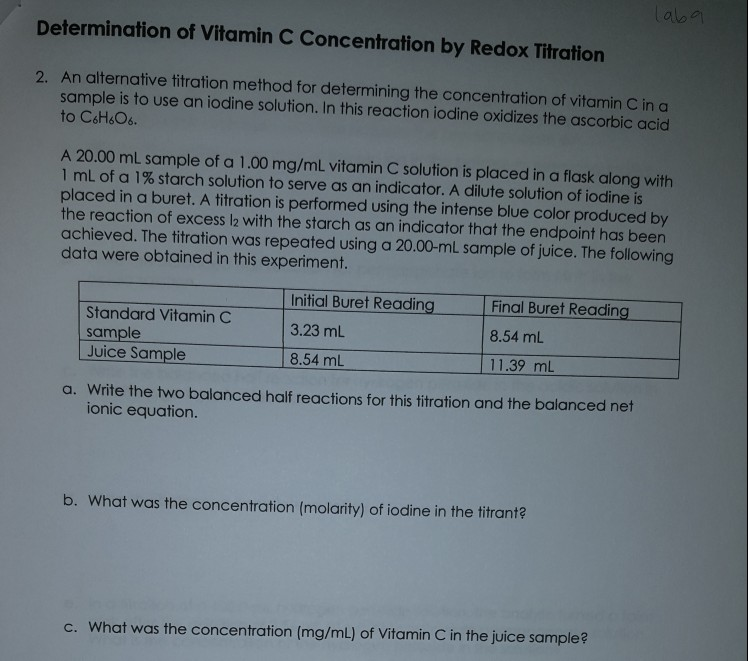Determination of Vitamin C Concentration by Redox Titration An alternative titration method for determining the concentration of vitamin C in a sample is to use an iodine solution. In this reaction iodine oxidizes the ascorbic acid to C&H&O 2. A 20.00 ml sample of a 1.00 mg/mL vitamin C solution is placed in a flask along with 1 mL of a 1% starch solution to serve as an indicator. A dilute solution of iodine is placed in a buret. A...

• ### just need help with questions 3. a & b always rate!!! Introduction Titration is a common...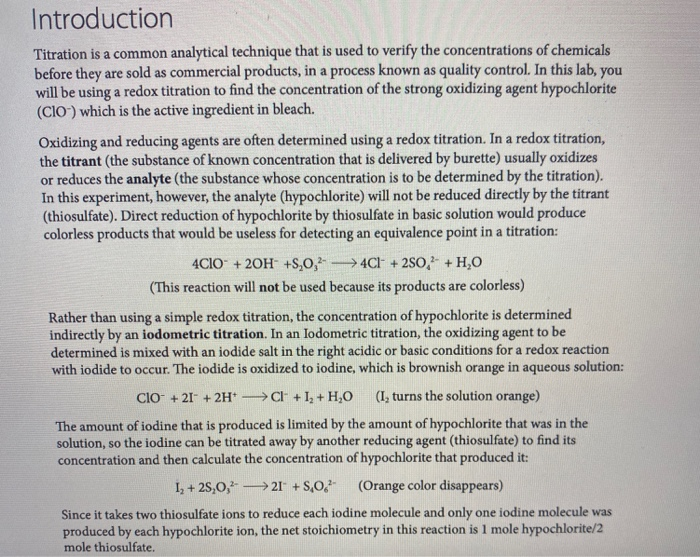just need help with questions 3. a & b always rate!!! Introduction Titration is a common analytical technique that is used to verify the concentrations of chemicals before they are sold as commercial products, in a process known as quality control. In this lab, you will be using a redox titration to find the concentration of the strong oxidizing agent hypochlorite (CIO) which is the active ingredient in bleach. Oxidizing and reducing agents are often determined using a redox titration....

• ### 16 7-2 Titration Calculations Ascorbic acid (vitamin C) reacts with according to the equation Starch is used as an i...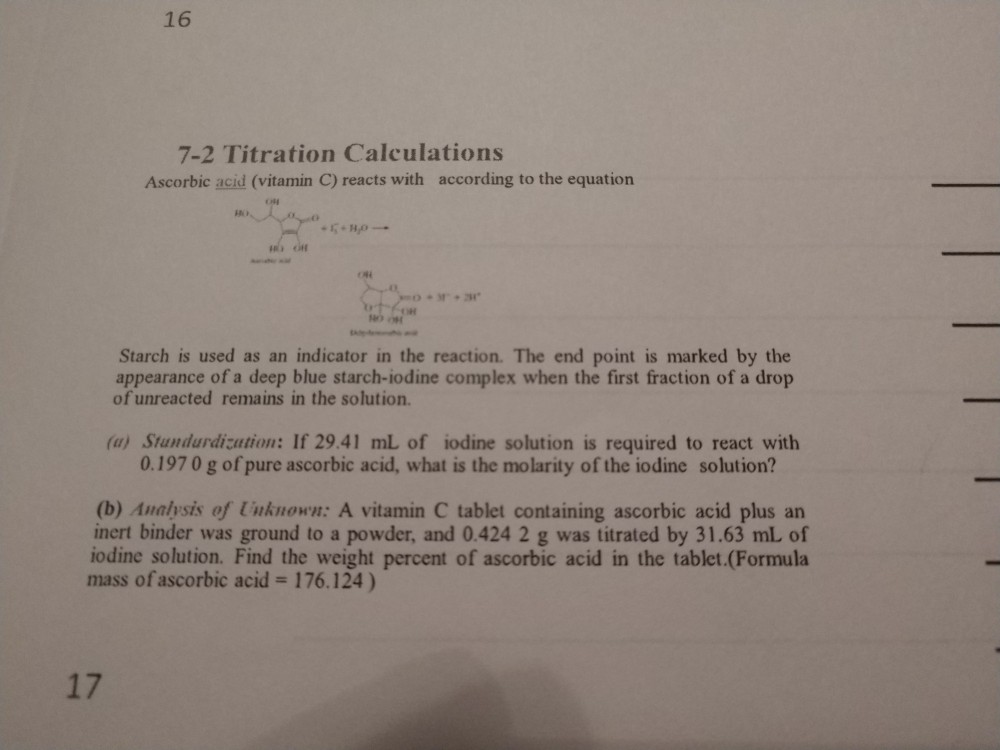16 7-2 Titration Calculations Ascorbic acid (vitamin C) reacts with according to the equation Starch is used as an indicator in the reaction. The end point is marked by the appearance of a deep blue starch-iodine complex when the first fraction of a drop of unreacted remains in the solution. TI (a) Stundurdization: If 29.41 mL of iodine solution is required to react with 0.197 0 g of pure ascorbic acid, what is the molarity of the iodine solution?! (b)...

• ### please I need help with this one question. Titration Procedure Prepare your starch solution by dissolving...

please I need help with this one question. Titration Procedure Prepare your starch solution by dissolving 0.040g of vitex starch in 20 mL of water. Prepare your 3%diluted commercial bleach solution (3% of the concentration of the commercial bleach, by volume.) Do not discard as you will use this solution for each of your trials. Dependingonyourinstructor’schosenmethod;deliverexactly3mLofcommercialbleach solution to a 100mL volumetric flask using the burette that contains the class’s supply of bleach, or pour some from the bottle into a...

• ### -50 ml burette -Titrant Stopcock -250 mL Erlenmeyer flask -Analyte CH Figure 2. Burettes are calibrated...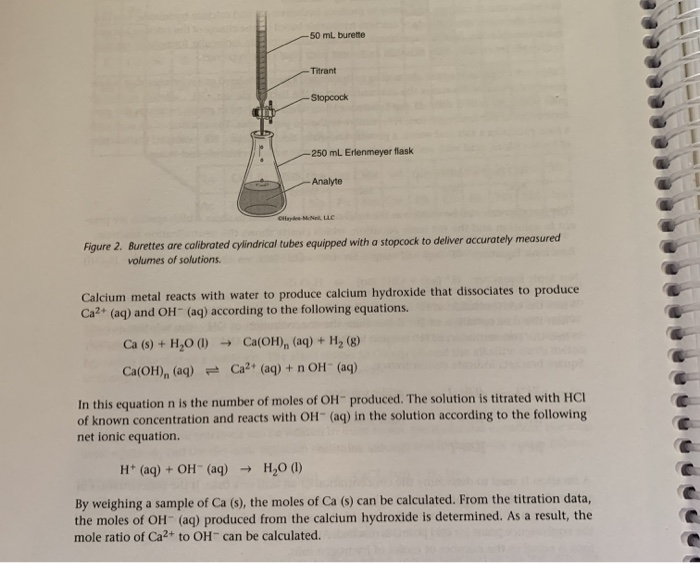-50 ml burette -Titrant Stopcock -250 mL Erlenmeyer flask -Analyte CH Figure 2. Burettes are calibrated cylindrical tubes equipped with a stopcock to deliver accurately measured volumes of solutions Calcium metal reacts with water to produce calcium hydroxide that dissociates to produce Ca? (aq) and OH(aq) according to the following equations. Ca (s) + H2O (1) Ca(OH), (aq) = Ca(OH), (aq) + H(8) Ca2+ (aq) + n OH(aq) In this equation n is the number of moles of OH produced....

• ### How do I calculate the isolated moles and molarity of the 0.2M KIO3?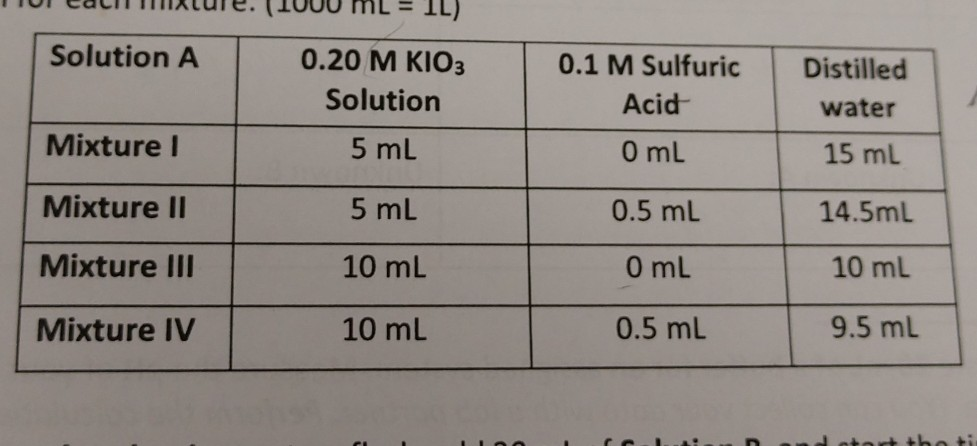how do I calculate the isolated moles and molarity of the 0.2M KIO3? 0.1 M Sulfuric Distilled Solution A Mixturel Mixture II Mixture III Mixture IV 0.20 M KIO3 Solution 5 mL 5 mL 10 mL 10 mL Acid 0 mL 0.5 mL 0 mL 0.5 mL water 15 ml 14.5mL 10 mL 9.5 mL Lalculations: I. (6pt) Calculate the total number of moles of iodate ion present in each 0.2 M KlO3 solution. Calculate the molarity of iodate ion...

• ### please answer these questions below Experiment 7: Titration II volume of deionized water so that(20 mL...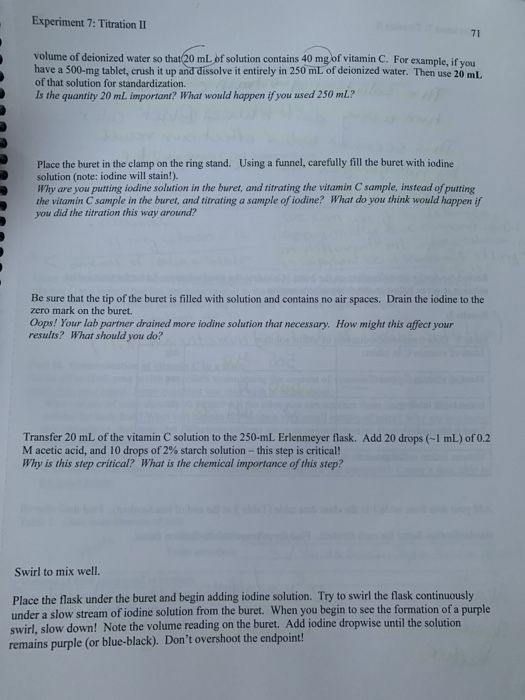please answer these questions below Experiment 7: Titration II volume of deionized water so that(20 mL of solution contains 40 mg of vitamin C. For example, if you have a 500-mg tablet, crush it up and dissolve it entirely in 250 mL of deionized water. Then use 20 mL of that solution for standardization. Is the quantity 20 mL important? What would happen if you used 250 mL? Place the buret in the clamp on the ring stand. Using a...